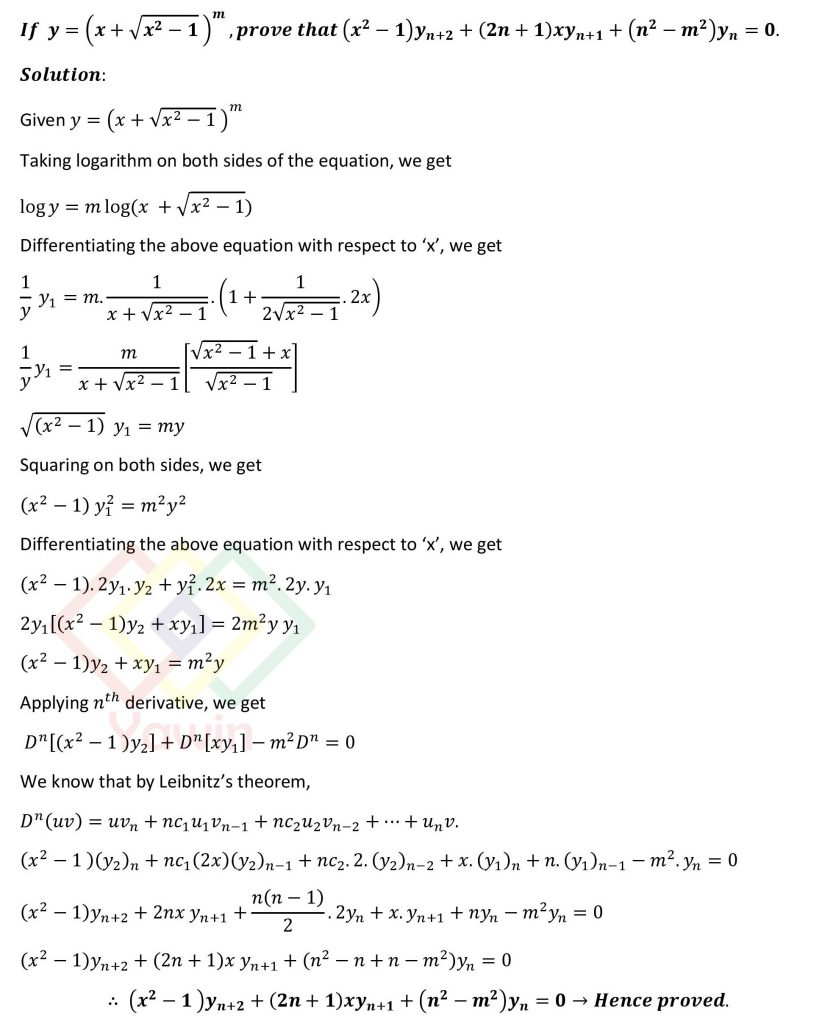If y=(x+sqrt(x^2-1))^m, prove that (x^2-1) y_(n+2)+(2n+1)xy_(n+1)+(n^2-m^2 ) y_n=0.

## Question## Question

If y=(x+sqrt(x^2-1))^m, prove that (x^2-1) y_(n+2)+(2n+1)xy_(n+1)+(n^2-m^2 ) y_n=0.

## Topic

Leibnitz’s theorem